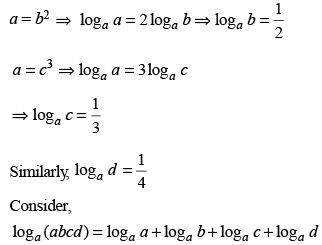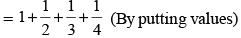Courses

# Test: Algebra - 2

## 25 Questions MCQ Test Topic-wise Past Year Questions for CAT | Test: Algebra - 2

Description
This mock test of Test: Algebra - 2 for CAT helps you for every CAT entrance exam. This contains 25 Multiple Choice Questions for CAT Test: Algebra - 2 (mcq) to study with solutions a complete question bank. The solved questions answers in this Test: Algebra - 2 quiz give you a good mix of easy questions and tough questions. CAT students definitely take this Test: Algebra - 2 exercise for a better result in the exam. You can find other Test: Algebra - 2 extra questions, long questions & short questions for CAT on EduRev as well by searching above.
QUESTION: 1

### If a1, a2, ... are in A.P., then,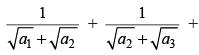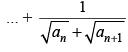is equal to (2019)

Solution:

As a1, a2, a3 ... are in A.P.
then,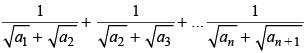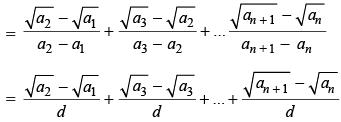{Where d = Common difference}.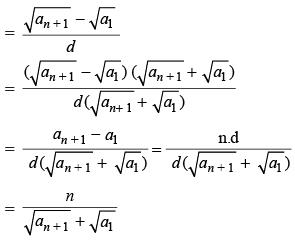QUESTION: 2

### Let x and y be positive real numbers such that log5 (x + y) + log5 (x – y) = 3, and log2 y – log2 x = 1 – log2 3. Then xy equals (2019)

Solution:

Given, log5(x + y) + log5(x – y) = 3
⇒ log5[(x + y)(x – y)] = 3
⇒ (x + y)(x – y) = 53 = 125
⇒ x2 – y2 = 125 ...(i)
And log2y – log2x = 1 – log2 3.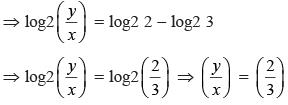Then if x = 3k, then y = 2k
Now putting this value of x and y in (i), we get
(3k)2 – (2k)2 = 125
⇒ 5k2 = 125, ∴ k = 5
∴ x × y = 3k × 2k = 6 × 25 = 150

QUESTION: 3

### If the population of a town is p in the beginning of any year then it becomes 3 + 2p in the beginning of the next year. If the population in the beginning of 2019 is 1000, then the population in the beginning of 2034 will be (2019)

Solution:

We can transform each of the options for ‘n’ years.
(997)214 + 3 ≡ (p – 3)2n – 1 + 3
(997)15 – 3 ≡ (p – 3)n – 3
(1003)15 + 6 ≡ (p + 3)n + 6
(1003)215 – 3 ≡ (p + 3)2n – 3
As per the condition, in one year, the population ‘p’ becomes ‘3 + 2p’
Putting the value of n = 1 in each option, and checking to get 3 + 2p,
we have (997)214 + 3 ≡ p ≠ 3 + 2p
(1003)15 + 6 ≡ p + 9 ≠ 3 + 2p
(1003)215 – 3 ≡ (p + 3)2n – 3 = 3 + 2p
(997)15 – 3 ≡ (p – 3) – 3 ≡ p – 6

QUESTION: 4

If (2n + 1) + (2n + 3) + (2n + 5) + ... + (2n + 47) = 5280, then what is the value of 1 + 2 + 3 + ... + n?

(2019)

Solution:

The sequence (2n + 1) + (2n + 3) + (2n + 5) + ... + (2n + 47) = 5280 is in A.P. with first term (a) = 2n + 1
common difference (d) = 2 and last term (l) = 2n + 47,
Let ‘m’ be the number of terms in this sequence, then
l = a + (m – 1)d
2n + 47 = (2n + 1) + (m – 1) (2) ⇒ m = 24
Now, (2n + 1) + (2n + 3) + (2n + 5) +....+ (2n + 47) = 5280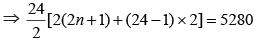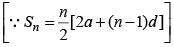⇒ 24(2n + 1 + 23) = 48(n + 12) = 5280
⇒ 48(n + 12) = 5280
∴ n = 98 Now, 1 + 2 + 3 + ... +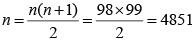QUESTION: 5

Let A be a real number. Then the roots of the equation x2 – 4x – log2A = 0 are real and distinct if and only if

(2019)

Solution:

We know that the quadratic equation ax2 + bx + c = 0 has real and distinct roots, if b2 – 4ac > 0 Hence for real and distinct roots of
x2 – 4x – log2 A = 0, D > 0
∴ (–4)2 – 4 × 1 × (–log2A) > 0
⇒ 16 + 4 log2A > 0 ⇒ log2A > – 4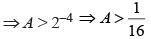QUESTION: 6

If x is a real number, then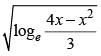is a real number if and only if

Solution:

The given expression will be real only if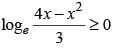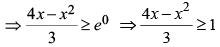⇒ 4x – x2 ≥ 3 ⇒ x2 – 4x + 3 ≤ 0
⇒ (x – 1) (x – 3) ≤ 0 ⇒ 1 ≤ x ≤ 3

QUESTION: 7

Let a1, a2, ... be integers such that a1 – a2 + a3 – a4 + ... + (–1)n–1. an = n, for all n ≥ 1.
Then a51 + a52 + . . . + a1023 equals

(2019)

Solution:

For n = 1 , a1 = 1
For n = 2 , a1 – a2 = 2 ⇒ a2 = –1
For n = 3 , a1 – a2 + a3 = 3 ⇒ a3 = 1
For n = 4 , a1 – a2 + a3 – a4 = 4 ⇒ a4 = –1
From the above shown pattern, we conclude that each odd term = 1 and each even term = –1
⇒ a51 + a52 + ... + a1022 + a1023
= (a51 + a52 + ....+ a1022) + a1023
= 0 + 1 = 1

QUESTION: 8

Let x, y, z be three positive real numbers in a geometric progression such that x < y < z. If 5x, 16y, and 12z are in an arithmetic progression then the common ratio of the geometric progression is

(2018)

Solution:

Since, 5x, 16y, 12z are in AP.
∴ 32y = 5x + 12z …(1)
∵ x, y, z are in GP
∴ y2 = xz ...(2)
Squaring both sides of (1), we get
1024y2 = 25x2 + 144z2 + 120xz
⇒ 1024xz = 25x2 + 144z2 + 120xz
⇒ 25x2+144z2 - 904xz = 0
⇒ 25x2 - 900xz - 4xz + 144 z2 = 0
⇒ 25x(x - 36z) - 4z(x - 36z) = 0
⇒ (25x - 4z) (x - 36z) = 0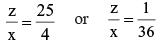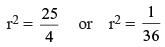[r is the common ratio]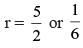But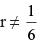because x, y, z > 0 and x < y < z
∴ common ratio = 5 / 2

QUESTION: 9

If x is a positive quantity such that 2x = 3log 52, then × is equal to

(2018)

Solution:

2x = 3log52
Taking logarithms to base 5 on both sides, we have
x (log52) = log52 . log53
x = log53 = log5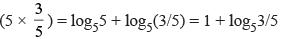QUESTION: 10

Given that X2018Y2017 = 1/2 and X2016Y2019 = 8, the value of x2 + y3 is

(2018)

Solution:

x2018 y2017 = 1/2 and x2016 y2019 = 8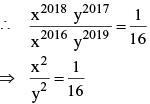⇒ y4035 = 24035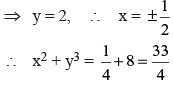QUESTION: 11

If log1281 = p, then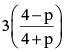is equal to

(2018)

Solution:

log1281 = p ⇒ log1234 = p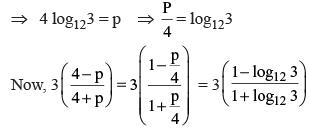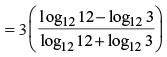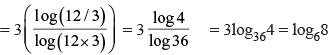QUESTION: 12

If log2(5 + log3 a) = 3 and log5(4a + 12 + log2 b) = 3, then a + b is equal to

(2018)

Solution:

5 + log3 a = 23 = 8 ⇒ log3a = 3  ⇒ a = 27 Similarly, 4a + 12 + log2b = 53 = 125
Since a = 27, 4(27) + 12 + log2b = 125
⇒ log2b = 5  ⇒ b = 32.
∴ a + b = 27 + 32 = 59

QUESTION: 13

Let a1, a2...., a2n be an arithmetic progression with a1 = 3 and a2 = 7. If a1 + a2 + ... + a3n = 1830, then what is the smallest positive integer m such that m(a1 + a2 + ... + an) > 1830?

(2017)

Solution:

a1 = 3, a2 = 7, d = 4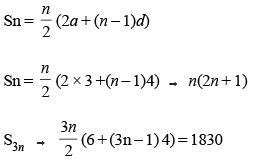n(6n + 1) = 610
6n2 + n - 610 = 0
solved, n = 10
Now, m(a1 + a2 + ... an) > 1830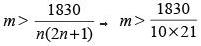m > 8.7 → m > 9

QUESTION: 14

If the square of the 7th term of an arithmetic progression with positive common difference equals the product of the 3rd and 17th terms, then the ratio of the first term to the common difference is

(2017)

Solution:

(a + 6d)2 = (a + 2d)(a + 16d)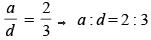QUESTION: 15

If 92x–1 – 81x–1 = 1944, then x is

(2017)

Solution:

92x–1 – 81x–1 = 1944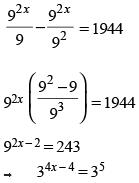4x – 4 = 5 → x = 9/4

QUESTION: 16

The value of log 0.008 √5 + log √3 81 - 7 is equal to

(2017)

Solution: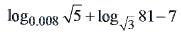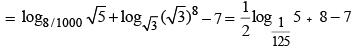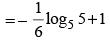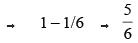QUESTION: 17

If x + 1 = x2 and x > 0, then 2x4 is

(2017)

Solution:

x2 – x – 1 = 0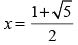(∵ x > 0)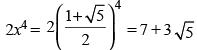QUESTION: 18

Two positive real numbers, a and b, are expressed as the sum of m positive real numbers and n positive real numbers respectively as follows:
a = s1 + s2 +…+ sm and b = t1 + t2 +…+ tn
If [a] = [s1] + [s2] +…+ [sm] + 4 and [b] = [t1 ] + [t2 ] +…+ [tn] + 3,
Where [x] denotes the greatest integer less than or equal to x, what is the minimum possible value of m + n?

(2016)

Solution:

If a positive number a is expressed as the sum of two positive numbers s1 and s2 then [a] could be at the most 1 more than [s1] + [s2], i.e., the fractional parts of s1 and s2 together, can provide at most 1.
Similarly, the fractional parts of s1, s2, s3, s4, s5 can together, provide at most 4.
Conversely, if [a] is 4 more than [s1] + [s2] + [s3] + [s4] + [sm], then m has to be at least 5.
Similarly, the least value of n is 4.
∴ (m + n)min = 5 + 4 = 9

QUESTION: 19

P1, P2, P3, ..., P11 are 11 friends. The number of balls with P1 through P11 in that order is in an Arithmetic Progression. If the sum of the number of balls with P1, P3, P5, P7, P9 and P11 is 72, what is the number of balls with P1, P6 and P11 put together?

(2014)

Solution:

Let the number of balls with
Pi = ai (i = 1 to 11)
a1 + a3 + a5 ...... + a11 = 6 (a6) = 72.
As a6 would be the arithmetic mean of these 11 numbers and
2(a6) = (a1 + a11)
= (a2 + a10)
= (a3 + a9)
= (a4 + a8)
= (a5 + a7)
∴ a1 + a6 + a11 = 3 (a6)
= 36

QUESTION: 20

If log32, log3(2x – 5) and log3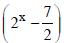are in Arithmetic Progression, then x is equal to

(2014)

Solution:

According to question,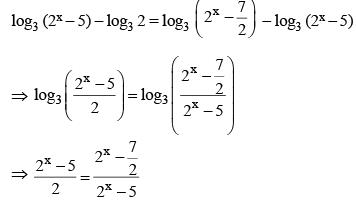Let 2x = a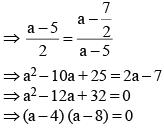⇒ a = 4 or 8
∴ x = 2 or 3
Hence, 2x – 5 = –1,
when x = 2, which is not possible.
∴ x = 3.
Or
All numbers are in AP,
2 log3 (2x – 5) = log3 2 + log3 (2x – 7/2)
Suppose 2x = t
2 log3 (t – 5) = log3 2 + log3 (t – 7/2)
→ log3 (t – 5)2 = log3 (2t – 7)
→ (t – 5)2 = 2t – 7
→ t2 – 10t + 25 = 2t – 7
→ t2 – 12t + 32 = 0
→ t = 4.8
2x = 4.8
∴ x = 2, 3.

QUESTION: 21

A ray of light along the line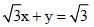gets reflected on the x-axis to become a ray along the line

(2014)

Solution:

Take –y in place of y
Then equation is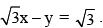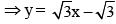QUESTION: 22

If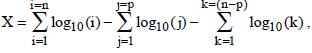where p ≤ n, then the maximum value of X for n = 8 is :

(2014)

Solution:

X = (log10 1 + log10 2 + ... + log10 n) – (log10 1 + log10 2 + .... + log10 p) – (log10 1 + log10 2 + .... + log10 (n – p))
⇒ X = log10 n! – log10 p! –  log10 (n – p)!
log (m × n) = log m + log n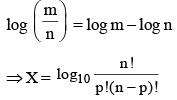X is maximum when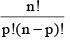is maximum.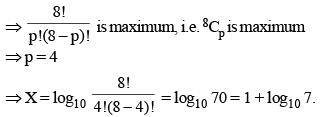QUESTION: 23

If x + y = 1, then what is the value of (x3 + y3 + 3xy)?

(2012)

Solution:

Given that x + y = 1
⇒ x + y – 1 = 0
⇒ x3 + y3 – 1 = – 3xy
(a3 + b3  + c3 = 3abc if a + b + c = 0)
⇒ x3 + y3 + 3xy = 1

QUESTION: 24

If log165 = m and log53 = n, then what is the value of log36 in terms of ‘m’ and ‘n’?

(2011)

Solution: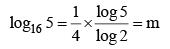...(i)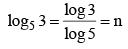...(ii)
From equations (i) and (ii), we get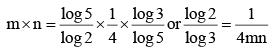Let log36 be equal to k; therefore,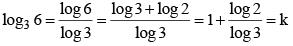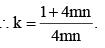QUESTION: 25

If a = b2 = c3 = d4 then the value of loga (abcd) would be :

(2010)

Solution:

Let a = b2 = c3 = d4